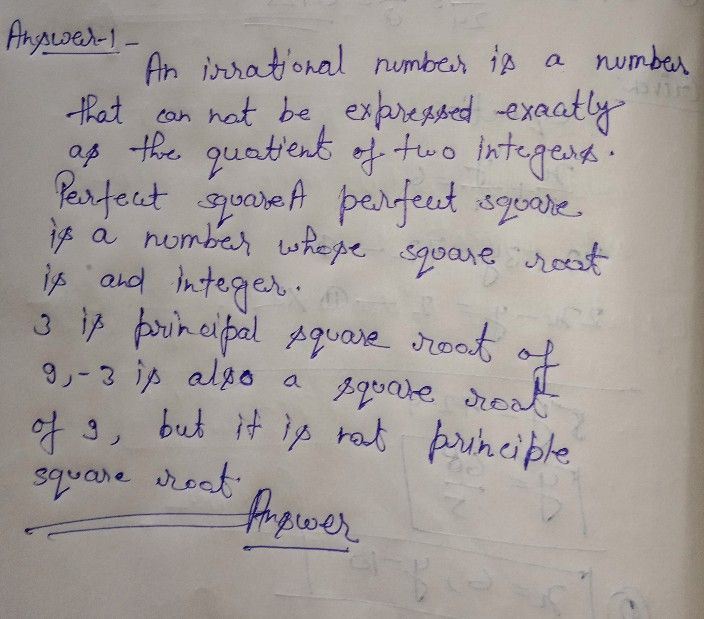Symbol
Problemior ON TAY After going through this module, you are expected $to:$ $1.$ $1\square$ Describe principal roots and tells whether they are rational or irrational. $2.I$ Determine between what two integers the square $To01$ of a number is. $3.F$ Plot irrational numbers on the number line. la' Count the total number of squares of the chess board. Prove that it has a total of $204$ squares. $\bar{1n}$
7th-9th grade
Calculus
Search count: 105
SolutionQanda teacher - henrysee